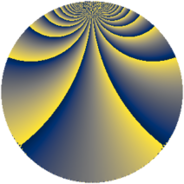# Properties

 Label 912.3.tLevel $912$ Weight $3$ Character orbit 912.t Rep. character $\chi_{912}(37,\cdot)$ Character field $\Q(\zeta_{4})$ Dimension $320$ Sturm bound $480$

# Related objects

## Defining parameters

 Level: $$N$$ $$=$$ $$912 = 2^{4} \cdot 3 \cdot 19$$ Weight: $$k$$ $$=$$ $$3$$ Character orbit: $$[\chi]$$ $$=$$ 912.t (of order $$4$$ and degree $$2$$) Character conductor: $$\operatorname{cond}(\chi)$$ $$=$$ $$304$$ Character field: $$\Q(i)$$ Sturm bound: $$480$$

## Dimensions

The following table gives the dimensions of various subspaces of $$M_{3}(912, [\chi])$$.

Total New Old
Modular forms 648 320 328
Cusp forms 632 320 312
Eisenstein series 16 0 16

## Trace form

 $$320q + O(q^{10})$$ $$320q + 56q^{16} - 32q^{19} - 72q^{24} - 200q^{26} + 120q^{28} - 192q^{35} + 24q^{36} + 112q^{38} + 280q^{44} - 2240q^{49} + 72q^{54} - 168q^{58} + 64q^{61} - 24q^{62} - 312q^{64} + 144q^{66} + 1016q^{68} - 520q^{74} - 384q^{76} - 328q^{80} - 2880q^{81} - 40q^{82} - 320q^{83} - 320q^{85} + 264q^{92} - 768q^{95} - 360q^{96} + O(q^{100})$$

## Decomposition of $$S_{3}^{\mathrm{new}}(912, [\chi])$$ into newform subspaces

The newforms in this space have not yet been added to the LMFDB.

## Decomposition of $$S_{3}^{\mathrm{old}}(912, [\chi])$$ into lower level spaces

$$S_{3}^{\mathrm{old}}(912, [\chi]) \cong$$ $$S_{3}^{\mathrm{new}}(304, [\chi])$$$$^{\oplus 2}$$